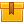## Prerequisites

This lesson is designed for participants familiar with the principles of statistical process control as well as control charts for variables and attributes. Knowledge of basic mathematical skills is recommended.

## Description

This lesson explains how to calculate the control limits for variable and attribute control charts. The lesson also shows how to construct and interpret cusum charts in order to improve process performance.

## Objectives

Calculate the standard deviation and control limits for mean variable control charts including mean and median range charts, and p, np, u, and c charts. Define local mean. Interpret a cusum chart for statistical control.

## ContentsThis lesson explains how to calculate the control limits for variable
and attribute control charts. The lesson also shows how to construct and
interpret cusum charts in order to improve process performance.

•Advanced Control Charts

0
0 Reviews